# The Matrix at the Heart of Computer Graphics

Matrices like the ones shown in the following screen shots are at the heart of computer graphics. They describe objects moving in three-dimensional space. MATLAB's Handle Graphics uses them. So does MathWork's new RoadRunner editor. And so do all popular video games and CAD packages.

### Contents

#### Utah Teapot

From its introduction in 1976 by Martin Newell, then a grad student at the University of Utah, the Teapot has played an import role in the development of computer graphics. If you knew to look for it, you could have seen it in Pixar's movies and on episodes of Fox TV's Simpsons. There are an excellent Wikipedia page and an interesting exhibit at the Computer History Museum. The coordinates of the teapot are available in MATLAB with the function teapotGeometry. A MATLAB live script describing shading and lighting of the teapot is available here.

   Teapot = grafix;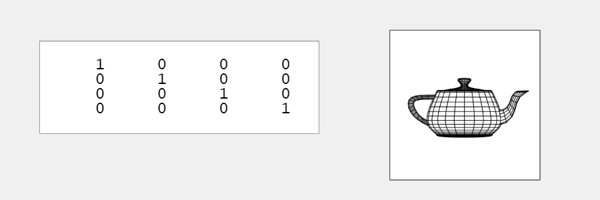#### M

I am interested in M, the matrix in the panel. It is now the 4-by-4 identity matrix. This matrix describes all the transformations made on a collection of target objects.

#### Rotation

Homogeneous coordinates make it possible to describe rotations, translations and many other transformations with 4-by-4 matrices. The matrices operate on vectors with the coordinates of the objects in the first three components and, for now, a one in the fourth.

This matrix leaves the z coordinates fixed while rotating x and y.

   R_z(t) = [ cosd(t)  -sind(t)         0        0
sind(t)   cosd(t)         0        0
0         0         1        0
0         0         0        1]

Here is a 45 degree rotation about z.

    Rz = R_z(45)

Rz =

0.7071   -0.7071         0         0
0.7071    0.7071         0         0
0         0    1.0000         0
0         0         0    1.0000



Apply this rotation to the teapot.

    apply(Teapot,Rz)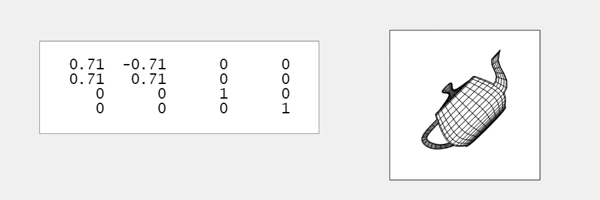#### Rz*Rx

A sequence of transformations is represented by the product of all the matrices.

This orthogonal rotation leaves x fixed while rotating y and z.

   R_x(t) = [       1         0         0        0
0   cosd(t)  -sind(t)        0
0   sind(t)   cosd(t)        0
0         0         0        1]

Follow our original 45 degree z transformation by a 60 degree rotation about x.

    Rx = R_x(60)
M = Rx*Rz;
apply(Teapot,M)

Rx =

1.0000         0         0         0
0    0.5000   -0.8660         0
0    0.8660    0.5000         0
0         0         0    1.0000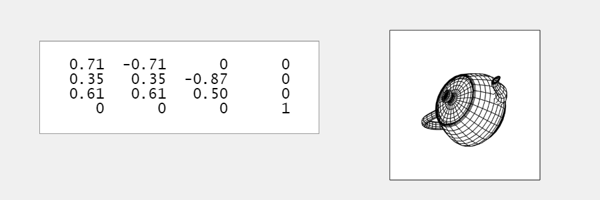The order is important because these matrices do not commute. Here is the result of reversing the order.

    M = Rz*Rx;
apply(Teapot,M)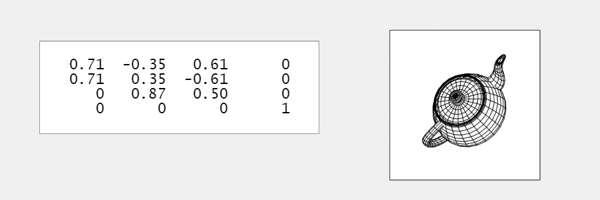#### Ry

This leaves y fixed while rotating x and z.

   R_y(t) = [ cosd(t)         0  -sind(t)        0
0         1         0        0
sind(t)         0   cosd(t)        0
0         0         0        1]
    Ry = R_y(-120)
apply(Teapot,Ry)

Ry =

-0.5000         0   -0.8660         0
0    1.0000         0         0
0.8660         0   -0.5000         0
0         0         0    1.0000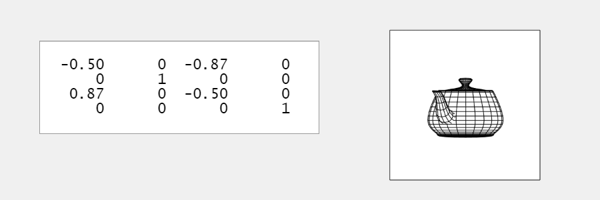#### Translation and scaling

Nonorthogonal matrices come from translation and scaling. Homogeneous coordinates and the fourth column of M produce translation. A typical translation is

   T_x(t) = [ 1   0   0   t
0   1   0   0
0   0   1   0
0   0   0   1];
    Tx = T_x(2.5)

Tx =

1.0000         0         0    2.5000
0    1.0000         0         0
0         0    1.0000         0
0         0         0    1.0000



Translate the teapot along the x axis.

    apply(Teapot,Tx)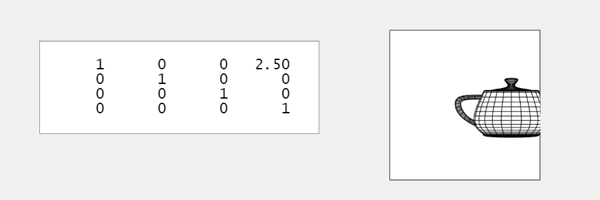We can scale each coordinate direction individually. Or, this matrix applies one factor to all of the coordinates.

   S_xyz(t) = [ t   0   0   0
0   t   0   0
0   0   t   0
0   0   0   1];
    Sxyz = S_xyz(0.75)

Sxyz =

0.7500         0         0         0
0    0.7500         0         0
0         0    0.7500         0
0         0         0    1.0000



"Honey, I shrunk the teapot".

    apply(Teapot,Sxyz)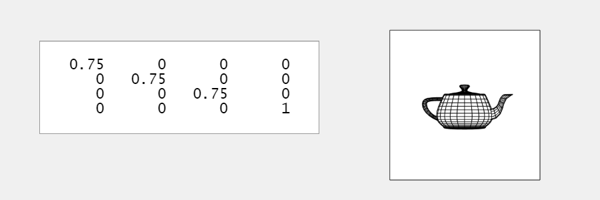#### All together

I have generated five transformations, Rx, Rz, Ry, Tx and Sxyz. Here is their product, in the order of their introduction.

    M = Sxyz*Tx*Ry*Rz*Rx;
apply(Teapot,M)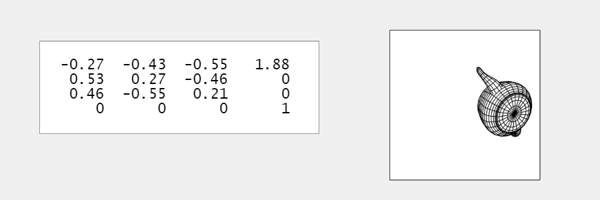Here is the reverse order.

    M = Rx*Rz*Ry*Tx*Sxyz;
apply(Teapot,M)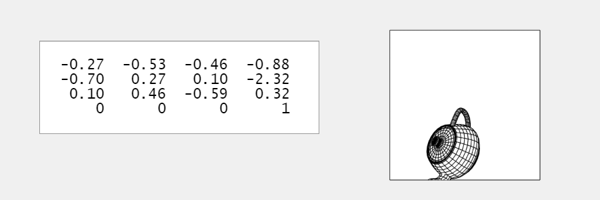Finally, a scrambled order.

    M = Rz*Ry*Sxyz*Tx*Rx;
apply(Teapot,M)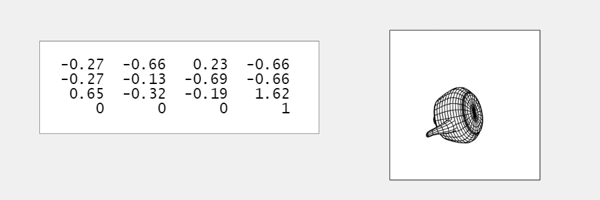Published with MATLAB® R2021b

|